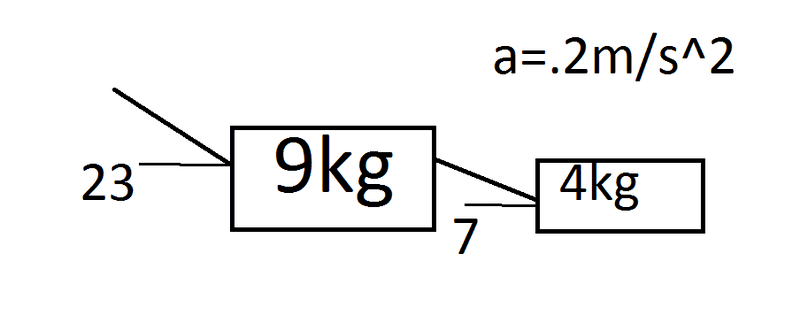# Two boxes connected by strings at an angle

## Homework Statement

Two boxes, A & B are on a flat surface, and are connected by a massless string at an angle of 7° to the horizontal. Box A masses 9kg, box b 4kg. The boxes are pulled by a string connected to box A at an angle of 23° to the horizontal. Find the force required in order to achieve an acceleration of .2m/s^2. Assume no frictional force.Fnet = m(a)

## The Attempt at a Solution

So far I've figured out the tension force on box A in the horizontal direction to be Ftx = Ftcos23°.
Normally, I would just multiply m(a), in this case 13(.2) to find 2.8N required in the horizontal direction. Given that Ftx = Ftcos23°, I would think to divide by cos23°, but this results in a negative answer. I'm also unsure as to how the boxes being connected by an angled string affects this.

EDIT: The negative answer was a calculation error, I'm getting 3.04N now.

Last edited:

Yes, except 13(.2) = 2.6 N.